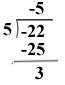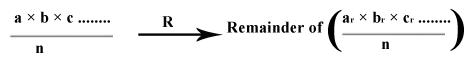# Concept of Remainder

Hello guys! Today we will discuss about concept of remainder and other methods to find remainder of a number.

Firstly tell me what will be the remainder when number 22 is divided by 5. Yes, as you guess it will be 2 as depicted below:Now what will be the remainder when -22 is divided by 5? Many of you might guess it will be -2, but you are wrong. So let’s see how:It is 3.

Note: Remainder is always non-negative. It cannot be negative.
Rule: Dividend = Divisor × Quotient + Remainder## Remainder Theorem

It states that remainder  of product of number is such that:Where ar = remainder when a is divided by n,
br = remainder when b is divided by n,
cr = remainder when c is divided by n.
Problems:
1.       Find remainder of (32×28×21)/6
(32×28×21)/6 à (2×4×3)/6 à24/6 à 0
Remainder is 0.
2.       Find remainder of (51×61×71)/4
(51×61×71)/4 à (3×1×3)/4 à9/4 à 1
Remainder is 1.
Similarly for addition, the method for calculating the remainder is same as given below:

### Important Rules to find the remainder

Rule 1:  For ‘a’ and ‘n’ be any position integer
1. Remainder of an/(a+1) is ‘a‘ i.e.
an/(a+1) à a, if ‘n’ is odd.
2. Remainder of an/(a+1) is ‘1‘ i.e.
an/(a+1) à 1, if ‘n’ is even.
Problems:
1. Remainder of 2100/3 = 1.
2. Remainder of 399/4 = 3.
3. Remainder of 65205/66 = 65.
4. Find remainder of (65206+1)/66
(65206+1)/66 à (1+1)/66 à2
Rule 2: For ‘a’ and ‘n’ be any position integer
1. Remainder of (ax+b)n/a is remainder of bn/a
(ax+b)n/a àremainder of bn/a
2. Remainder of (ax+1)n/a is 1
(ax+1)n/a àremainder of bn/a
Problems:
1. Find the remainder of 51203/7
Solution: 51203/7 = (7×7+2)203/7 à 2203/7 = ((23)67×22)/7 à (867×4)/7 à ((7×1+1)67×4)/7 à (1×4)/7 à 4